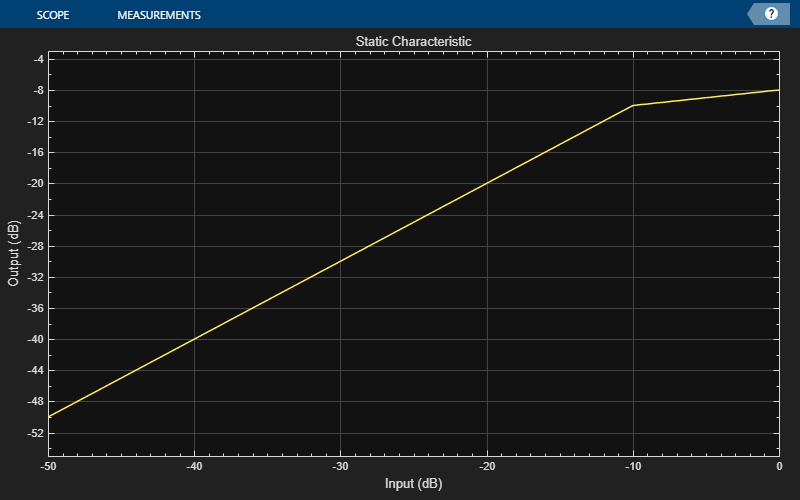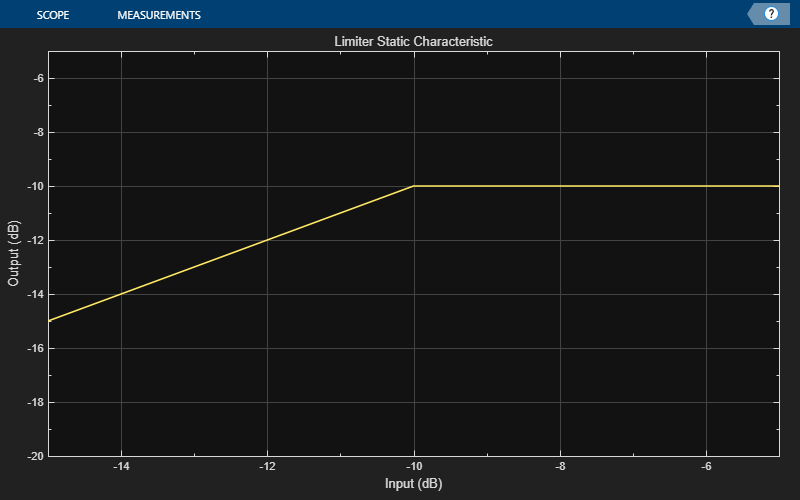# visualize

Visualize static characteristic of dynamic range controller

## Syntax

``visualize(dynamicRangeController)``
``visualize(dynamicRangeController,inputRange)``
``hvsz = visualize(___)``

## Description

example

````visualize(dynamicRangeController)` plots the static characteristic of the dynamic range control object. The plot is updated automatically when properties of the object change.```

example

````visualize(dynamicRangeController,inputRange)` enables you to specify the input range.```

example

````hvsz = visualize(___)` returns a handle to the visualizer when called with any of the previous syntaxes.```

## Examples

collapse all

Create a `compressor` System object™, and then plot the static characteristic.

```dynamicRangeCompressor = compressor; visualize(dynamicRangeCompressor)```The static characteristic plot updates automatically if you modify a property of the object.

`dynamicRangeCompressor.Threshold = -30;`Create an `expander` System object™. Plot the static characteristic over the range –15 to –5, in 0.001 dB increments.

```dynamicRangeExpander = expander; visualize(dynamicRangeExpander,-15:0.001:-5)```Create a `limiter` System object™. Get the output level of the static characteristic over a specified range.

```dynamicRangeLimiter = limiter; inputLevel = -15:1:-5```
```inputLevel = 1×11 -15 -14 -13 -12 -11 -10 -9 -8 -7 -6 -5 ```
`outputLevel = staticCharacteristic(dynamicRangeLimiter,inputLevel)`
```outputLevel = 1×11 -15 -14 -13 -12 -11 -10 -10 -10 -10 -10 -10 ```

Plot the static characteristic. Modify the title to state that the object is a limiter.

```hvsz = visualize(dynamicRangeLimiter,inputLevel); hvsz.Title = "Limiter Static Characteristic";```## Input Arguments

collapse all

Dynamic range control object, specified as a `compressor`, `expander`, `limiter`, or `noiseGate` System object™.

Range over which to calculate the output of the static characteristic, specified as a vector of monotonically increasing values expressed in dB. The default input range is `[-50:0.01:0]` dB.

## Output Arguments

collapse all

Visualizer handle, returned as a `dsp.ArrayPlot` object.

## Version History

Introduced in R2016a

expand all

Behavior changed in R2022a

Behavior changed in R2022a